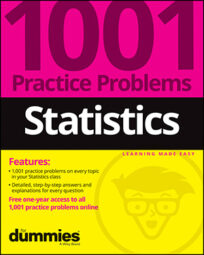##### Statistics: 1001 Practice Problems For Dummies (+ Free Online Practice)The empirical rule in statistics states that for a normal distribution, almost all data will fall within three standard deviations of the mean. Use the empirical rule to solve the following problems.

## Sample questions

1. According to the empirical rule (or the 68-95-99.7 rule), if a population has a normal distribution, approximately what percentage of values is within one standard deviation of the mean?

The empirical rule states that in a normal (bell-shaped) distribution, approximately 68% of values are within one standard deviation of the mean.

2. If the average age of retirement for the entire population in a country is 64 years and the distribution is normal with a standard deviation of 3.5 years, what is the approximate age range in which 95% of people retire?

The empirical rule states that in a normal distribution, 95% of values are within two standard deviations of the mean. "Within two standard deviations" means two standard deviations below the mean and two standard deviations above the mean.

In this case, the mean is 64 years, and the standard deviation is 3.5 years. So two standard deviations is (3.5)(2) = 7 years.

To find the lower end of the range, subtract two standard deviations from the mean: 64 – 7 years = 57 years. And then to find the upper end of the range, add two standard deviations to the mean: 64 + 7 years = 71 years.

So about 95% of people who retire do so between the ages of about 57 to 71 years.

3. What is a necessary condition for using the empirical rule (or 68-95-99.7 rule)?

Answer: if a population has a normal distribution

You can use the empirical rule only if the distribution of the population is normal. Note that the rule says that if the distribution is normal, then approximately 68% of the values lie within one standard deviation of the mean, not the other way around. Many distributions have 68% of the values within one standard deviation of the mean that don't look like a normal distribution.

4. The quality control specialists of a microscope manufacturing company test the lens for every microscope to make sure the dimensions are correct. In one month, 600 lenses are tested.

The mean thickness is 2 millimeters. The standard deviation is 0.000025 millimeters. The distribution is normal. The company rejects any lens that is more than two standard deviations from the mean. Approximately how many lenses out of the 600 would be rejected?

If you assume that the 600 lenses tested come from a population with a normal distribution (which they do), you can apply the empirical rule (also known as the 68-95-99.7 rule).

Using the empirical rule, approximately 95% of the data lies within two standard deviations of the mean, and 5% of the data lies outside this range. Because the lenses that are more than two standard deviations from the mean are rejected, you can expect about 5% of the 600 lenses, or (0.05)(600) = 30 lenses to be rejected.

5. Biologists gather data on a sample of fish in a large lake. They capture, measure the length of, and release 1,000 fish.

They find that the standard deviation is 5 centimeters, and the mean is 25 centimeters. They also notice that the shape of the distribution (according to a histogram) is very much skewed to the left (which means that some fish are smaller than most of the others). Approximately what percentage of fish in the lake is likely to have a length within one standard deviation of the mean?

Answer: cannot be determined with the information given

You could use the empirical rule (also known as the 68-95-99.7 rule) if the shape of the distribution of fish lengths was normal; however, this distribution is said to be "very much skewed left," so you can't use this rule. With the information given, you can't answer the question.

If you need more practice on this and other topics from your statistics course, visit 1,001 Statistics Practice Problems For Dummies to purchase online access to 1,001 statistics practice problems! We can help you track your performance, see where you need to study, and create customized problem sets to master your stats skills.